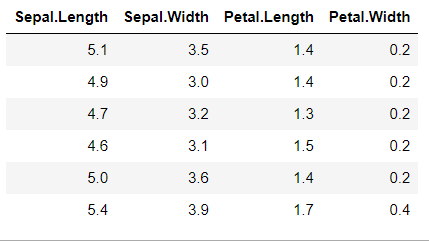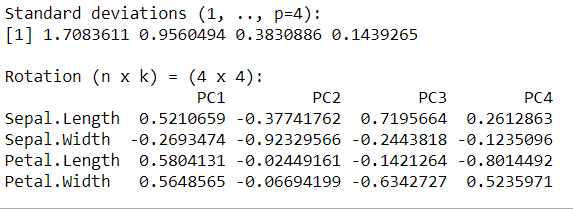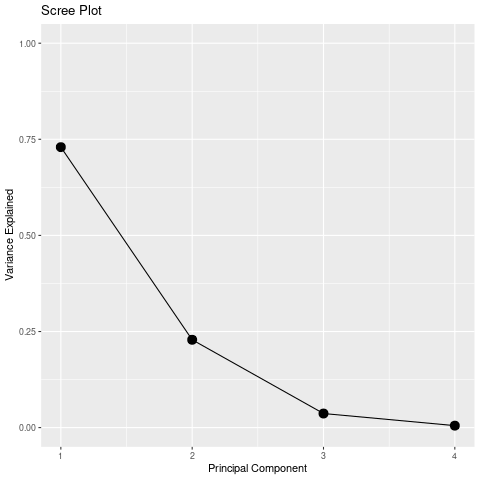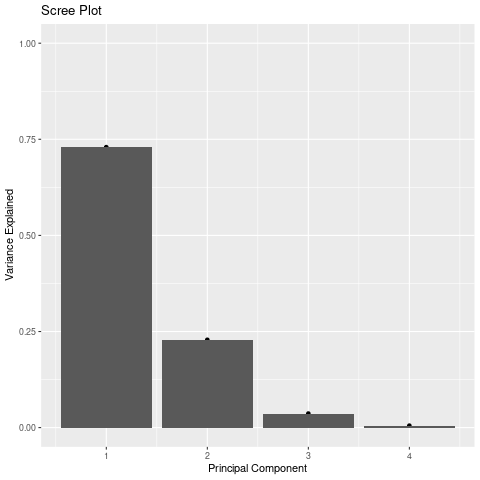How To Make Scree Plot in R with ggplot2

• Last Updated : 23 Sep, 2021

In this article, we are going to see how can we plot a Scree plot in R Programming Language with ggplot2

Here we will load the dataset, (Remember to drop the non-numerical column). Since the iris flower dataset contains a species column that is of character type so we need to drop it because PCA works with only numerical data.

R

 # drop the species column as its character typenum_iris = subset(iris,                  select = -c(Species))head(num_iris)

Output:Compute Principal Component Analysis using prcomp() function

We use R language’s inbuilt prcomp() function, this function takes the dataset as an argument and computes the PCA. Principal Component Analysis (PCA) is a statistical procedure that uses an orthogonal transformation that converts a set of correlated variables to a set of uncorrelated variables. Doing scale=TRUE standardizes the data.

Syntax: prcomp(numeric_data, scale = TRUE)

Code:

R

 # drop the species column as its character typenum_iris = subset(iris, select = -c(Species) ) # compute pcapca <- prcomp(num_iris, scale = TRUE)pca

Output:Compute variance explained by each Principal Component:

We use the formula below to compute the total variance experienced by each PC.

Syntax: pca\$sdev^2 / sum(pca\$sdev^2)

Code:

R

 # drop the species column as its character typenum_iris = subset(iris, select = -c(Species) ) # compute pcapca <- prcomp(num_iris, scale = TRUE) # compute total variancevariance = pca\$sdev^2 / sum(pca\$sdev^2)variance

Output:

 0.729624454 0.228507618 0.036689219 0.005178709

R

 library(ggplot2) # drop the species column as its character typenum_iris = subset(iris, select = -c(Species) ) # compute pcapca <- prcomp(num_iris, scale = TRUE) # compute total variancevariance = pca \$sdev^2 / sum(pca \$sdev^2) # Scree plotqplot(c(1:4), variance) +  geom_line() +  geom_point(size=4)+  xlab("Principal Component") +  ylab("Variance Explained") +  ggtitle("Scree Plot") +  ylim(0, 1)

Output:R

 library(ggplot2) # drop the species column as its character typenum_iris = subset(iris, select = -c(Species) ) # compute pcapca <- prcomp(num_iris, scale = TRUE) # compute total variancevariance = pca \$sdev^2 / sum(pca \$sdev^2) # Scree plotqplot(c(1:4), variance) +  geom_col()+  xlab("Principal Component") +  ylab("Variance Explained") +  ggtitle("Scree Plot") +  ylim(0, 1)

Output:My Personal Notes arrow_drop_up